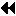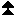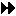An Application Example

# An Application ExampleUp: Process Topologies Next: MPI Environmental Management Previous: Low-level topology functions

```

The example in figure An Application Example
shows how the grid definition and
inquiry functions can be used in an application program. A partial
differential equation, for instance the Poisson equation, is to be
solved on a rectangular domain.
First, the processes organize themselves in a two-dimensional
structure. Each process then inquires about the ranks of its
neighbors in the four directions (up, down, right, left).
The numerical problem is solved by an iterative method, the details
of which are hidden in the subroutine  relax.

In each relaxation step each process computes new values for the solution grid
function at all points owned by the process. Then the values at inter-process
boundaries have to be exchanged with neighboring processes.  For example, the
exchange subroutine might contain a call like
MPI_SEND(...,neigh_rank(1),...) to send updated values to the
left-hand neighbor  (i-1,j).   ```

Set-up of process structure for two-dimensional parallel Poisson solver.Up: Process Topologies Next: MPI Environmental Management Previous: Low-level topology functions